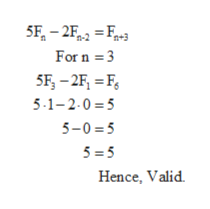# The Fibonacci sequence has many unusual properties. Experiment to decide which of the following properties are valid. Note: Fn represents the nth Fibonacci number.5Fn − 2Fn − 2 = Fn + 3   for n ≥ 3validnot valid

Question
8 views
The Fibonacci sequence has many unusual properties. Experiment to decide which of the following properties are valid. Note: Fn represents the nth Fibonacci number.
5Fn − 2Fn − 2 = Fn + 3   for n ≥ 3
valid
not valid
check_circle

Step 1

Consider the given series known as Fibonacci sequence. Series starts with 0, 1 and then each next term is written as the summation of previous two terms.

Hence, the most common sequence becomes 0, 1, 1, 2, 3, 5, 8, 13, 21…...so on

Step 2

We will check it in two ways, first let’s do it in short way using a small value of ‘n’.

Le...help_outlineImage Transcriptionclose5F, - 2F, = F+3 For n =3 5F, – 2F, = F, 5-1-2.0 =5 5-0 = 5 5 = 5 Hence, Valid. fullscreen

### Want to see the full answer?

See Solution

#### Want to see this answer and more?

Solutions are written by subject experts who are available 24/7. Questions are typically answered within 1 hour.*

See Solution
*Response times may vary by subject and question.
Tagged in